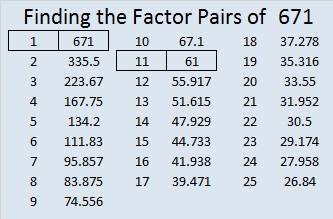# 671 is the Magic Sum of an 11 x 11 Magic Square

6 – 7 + 1 = 0 so 671 is divisible by 11.

671 is the sum of the fifteen prime numbers from 17 to 73.

Because 61 is one of its factors, 671 is the hypotenuse of the Pythagorean triple 121-660-671. The greatest common factor of those three numbers practically jumps out at me. Does it do the same thing to you?

Best of all 671 is the magic sum of an 11 x 11 magic square. (That link from wikipedia helped me construct this square. I’ll give directions so you can do it, too!)Notice how every row, column, and diagonal on the square sums to 671. The reason it is the magic sum is because the sum of all the numbers from 1 to 121 can be computed and then divided by 11 (the number of rows). Here is the equation:

• 671 = 121 x 120/2/11

Because 11 is an odd number there are simple directions to complete the entire square:

The number 1 is located in the exact center of the top row.

Find the number 2 on the square. (It’s located on the bottom row just right of the exact center square.) Notice that the numbers 3, 4, 5, and 6 are on the same diagonal. If you imagine the diagonal wrapping around the square, you can continue to follow it for numbers 7, 8, 9, 10, and 11. We can’t put the number 12 along the same diagonal because the number 1 is already in that spot, so we put the 12 UNDER the 11 and begin working on a new diagonal.

Anytime a number already occupies a space on a diagonal, put the next number under the preceding number and continue making a new diagonal. When a diagonal reaches the edge of the square, imagine that edge is connected to the opposite edge and continue the diagonal from the opposite edge.

I found it to be the trickiest placing the numbers 67 and 68, but other than that it was rather easy to know where to put the numbers.

Notice that the difference between any smaller number and the larger number just below it is either 12 or 1.

If you have excel on your computer, click on 12 Factors 2015-11-02, select the magic square tab, and then you can make this 11 x 11 magic square yourself. As you type in numbers, the columns, rows, and diagonals will automatically keep a running sum.

Once you get the square to give the magic sum in each direction, you can try doing the same thing with the 13 x 13 magic square that I’ve included on the same page. Its magic sum is 1105 which can be also be computed:

• 1105 = 169 x 170/22/13.

There is actually many more possible and probably more complicated 11 x 11 and 13 x 13 magic squares. I hope you enjoy making some with this easy method.

—————————————————————————————————

• 671 is a composite number.
• Prime factorization: 671 = 11 x 61
• The exponents in the prime factorization are 1 and 1. Adding one to each and multiplying we get (1 + 1)(1 + 1) = 2 x 2 = 4. Therefore 671 has exactly 4 factors.
• Factors of 671: 1, 11, 61, 671
• Factor pairs: 671 = 1 x 671 or 11 x 61
• 671 has no square factors that allow its square root to be simplified. √671 ≈ 25.903668.—————————————————————————————————

Advertisements

## 2 thoughts on “671 is the Magic Sum of an 11 x 11 Magic Square”

1.Joseph Nebus

I appreciate the instructions. I’d always supposed there were straightforward rules to making Magic Squares of arbitrary size but never did learn them.

•ivasallay

You’re welcome. I enjoyed learning the rules for making these odd numbered magic squares. It looks like there is also a pattern for squares with side lengths that are multiples of 4, but the pattern doesn’t look as easy.

This site uses Akismet to reduce spam. Learn how your comment data is processed.